SSC BOARD PAPERS IMPORTANT TOPICS COVERED FOR BOARD EXAM 2024

### Surface Area And Volume Class 9th Mathematics Part Ii MHB Solution

##### Surface Area And Volume Class 9th Mathematics Part Ii MHB Solution

###### Practice Set 9.1
Question 1.

Length, breadth, and height of a cuboid shape box of medicine are 20cm, 12 cm and 10 cm respectively. Find the surface area of vertical faces and total surface area of this box.

Given: A cuboid shape box of medicine having,

Length (l) = 20 cm

Height (h) = 10 cm

To find: Surface Area of vertical faces (SA) = ?

Total Surface Area of the box (cuboid) (TSA) = ?

To find Surface Area of vertical faces of cuboid:

It will be found by adding vertical sides of cuboid, i.e., front side, back side, left side and right side of the cuboid.

So, Surface Area of vertical faces = (Area of front face) + (Area of back face) + (Area of left face) + (Area of right face)

⇒ SA = lh + lh + bh + bh

⇒ SA = 2 lh + 2 bh

⇒ SA = 2(l + b)×h

⇒ SA = 2(20 + 12)×10

⇒ SA = 2 × 32 × 10

⇒ SA = 640

Thus, Surface Area of vertical faces of the box is 640 cm2.

To find Total Surface Area of the box:

We have a formula for Total Surface Area of cuboid given by,

TSA = 2(lb + bh + hl)

⇒ TSA = 2(20×12 + 12×10 + 10×20)

⇒ TSA = 2(240 + 120 + 200)

⇒ TSA = 2(560)

⇒ TSA = 1120

Thus, Total Surface Area of the box is 1120 cm2.

Hence, Surface Area of vertical faces of the box is 640 cm2 and Total Surface Area of the box is 1120 cm2.

Question 2.

Total surface area of a box of cuboid shape is 500 sq. unit. Its breadth and height are 6 unit and 5 unit respectively. What is the length of that box?

Given: Total surface area of cuboidal box (TSA) = 500 sq. unit

Breadth of cuboidal box (b) = 6 unit

Height of cuboidal box (h) = 5 unit

To find length (l) of the cuboidal box, recall total surface area of cuboid given by

TSA = 2 (lb + bh + hl)

Substituting given values in the formula, we get

500 = 2 (l×6 + 6×5 + 5×l)

⇒ 500 = 2 (6l + 30 + 5l)

⇒ 6l + 5l + 30 = 500/2

⇒ 11l + 30 = 250

⇒ 11l = 250 – 30 = 220

⇒ l = 220/11 = 20

Thus, length of the cuboidal box is 20 unit.

Question 3.

Side of a cube is 4.5 cm. Find the surface area of all vertical faces and total surface area of the cube.

Given: side of the cube (s) = 4.5 cm

We know that, cube has equal sides. This means, length = breadth = height.

So, to find surface area of all vertical faces of the cube, we need to add front side area, back side area, left side area and right-side area of the cube.

∴, Surface area (SA) = (Area of front face) + (Area of back face) + (Area of left face) + (Area of right face)

⇒ SA = s2 + s2 + s2 + s2 = 4s2

⇒ SA = 4(4.5)2

⇒ SA = 81

Also, Total surface area of cube is given by

TSA = 6 (side)2

⇒ TSA = 6 (s)2

⇒ TSA = 6 (4.5)2

⇒ TSA = 121.50

Thus, surface area of all vertical faces of the cube is 81 cm2 and total surface area of the cube is 121.50 cm2.

Question 4.

Total surface area of a cube is 5400 sq. cm. Find the surface area of all vertical faces of the cube.

Given: Total surface area of cube = 5400 cm2

And we know total surface area of cube is given by,

TSA = 6 (side)2

⇒ 5400 = 6 (side)2 [∵, it given that TSA = 5400 cm2]

⇒ (side)2 = 5400/6

⇒ (side)2 = 900

⇒ side = √900

⇒ side = 30

Now we know side = 30 cm.

Let side = s.

So, to find surface area of all vertical faces of the cube, we need to add front side area, back side area, left side area and right side area of the cube.

∴, Surface area (SA) = (Area of front face) + (Area of back face) + (Area of left face) + (Area of right face)

⇒ SA = s2 + s2 + s2 + s2 = 4s2

⇒ SA = 4 (30)2

⇒ SA = 3600

Thus, surface area of all vertical faces of the cube is 3600 cm2.

Question 5.

Volume of a cuboid is 34.50 cubic metre. Breadth and height of the cuboid is 1.5m and 1.15m respectively. Find its length.

Given: Volume of cuboid (V) = 34.50 m3

Breadth of cuboid (b) = 1.5 m

Height of cuboid (h) = 1.15 m

Volume of a cuboid is given by,

Volume = length × breadth × height

⇒ V = lbh

⇒ l = V/bh

⇒ l =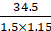⇒ l = 20

Thus, length of cuboid is 20 m.

Question 6.

What will be the volume of a cube having length of edge 7.5 cm?

Given: length of edge (s) = 7.5 cm

To find volume of cube, we need to write the formula of volume of cube:

Volume of cube (V) = (length of edge)3

⇒ V = (7.5)3 [from given]

⇒ V = 421.88

Thus, volume of the required cube is 421.88 cm3.

Question 7.

Radius of base of a cylinder is 20cm and its height is 13cm, find its curved surface area and total surface area.

(π = 3.14)

Given: radius of base of cylinder (r) = 20 cm

Height of cylinder (h) = 13 cm

Since it is a cylinder, curved surface area is given by

CSA = 2πrh

Where CSA = Curved Surface Area

h = height

⇒ CSA = 2 × 3.14 × 20 × 13

⇒ CSA = 1632.80 …(i)

Total surface area (TSA) of cylinder is given by

TSA = (Curved surface area) + 2 (Circular area of the base of the cylinder)

⇒ TSA = 2πrh + 2πr2

⇒ TSA = 1632.8 + (2 × 3.14 × (20)2) [from (i)]

⇒ TSA = 1632.8 + 2512 = 4144.80

Thus, curved surface area of the cylinder is 1632.80 cm2 and total surface area of the cylinder is 4144.80 cm2.

Question 8.

Curved surface area of a cylinder is 1980 cm2 and radius of its base is 15cm. Find the height of the cylinder.

(π = 22/7)

Given: Curved surface area of the cylinder (CSA) = 1980 cm2

Radius of the base of cylinder (r) = 15 cm

We need to write curved surface area of cylinder, that is

CSA = 2πrh

h = height of the cylinder

So by substituting values in the formula, we get

1980 = 2 × 22/7 × 15 × h

⇒ h =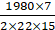⇒ h = 21

Thus, height of the cylinder is 21 cm.

###### Practice Set 9.2
Question 1.

Perpendicular height of a cone is 12 cm and its slant height is 13 cm. Find the radius of the base of the cone.

We have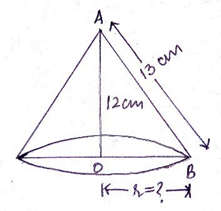Given that, height of cone = 12 cm and slant height of cone = 13 cm.

We need to find radius of the base of the cone, r.

In right-angled ∆AOB, using Pythagoras theorem,

AB2 = AO2 + OB2

⇒ OB2 = AB2 – AO2

⇒ r2 = 132 – 122

⇒ r2 = 169 – 144 = 25

⇒ r = √25

⇒ r = 5

Thus, radius of the base of the cone is 5 cm.

Question 2.

Find the volume of a cone, if its total surface area is 7128 sq.cm and radius of base is 28 cm.

(π = 22/7)

Given: Total surface area of cone (TSA) = 7128 cm2

Radius of base of cone (r) = 28 cm

We know total surface area of cone is given by,

TSA = πrl + πr2

⇒ πrl = TSA – πr2

⇒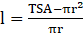⇒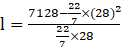⇒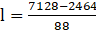⇒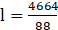⇒ l = 53

So, slant height of cone = 53 cm

We have,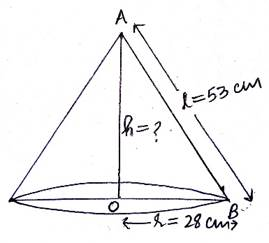Using Pythagoras theorem in ∆AOB, we can find height of the cone.

AB2 = AO2 + OB2

⇒ AO2 = AB2 – OB2

⇒ h2 = 532 – 282

⇒ h2 = 2809 – 784

⇒ h = √2025 = 45

We have got height (h) = 45 cm.

Volume of cone is given by,

V = πr2h/3

⇒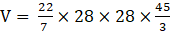⇒ V = 36960

Thus, volume of cone is 36960 cm3.

Question 3.

Curved surface area of a cone is 251.2 cm2 and radius of its base is 8cm. Find its slant height and perpendicular height. (π = 3.14)

We have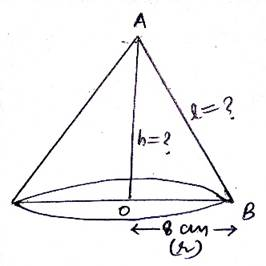Given: Curved surface area (CSA) = 251.2 cm2

Radius of the base of cone (r) = 8 cm

We know the formula of curved surface area of cone,

CSA = πrl

Where l = slant height of cone

⇒ 251.2 = 3.14 × 8 × l

⇒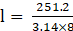⇒ l = 10

So, we have found slant height of cone (l) = 10 cm

Now, using Pythagoras theorem in ∆AOB,

AB2 = AO2 + OB2

⇒ AO2 = AB2 – OB2

⇒ h2 = 102 – 82

⇒ h2 = 100 – 64

⇒ h = √36 = 6

Thus, slant height of cone is 10 cm and height of cone is 6 cm.

Question 4.

What will be the cost of making a closed cone of tin sheet having radius of base 6 m and slant height 8 m if the rate of making is Rs.10 per sq.m?

Given: radius of base of cone (r) = 6 m

Slant height of cone (l) = 8 m

Since, this is a closed cone made of tin sheet. We need to find total surface area of the cone, it given by

TSA = πrl + πr2

⇒ TSA = πr (l + r)

Substituting values in the formula, we get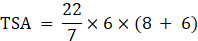⇒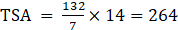According to the question,

Cost of 1 m2 = Rs. 10

Cost of 264 m2 = 10 × 264

= Rs. 2640

Thus, cost of making the required cone is Rs. 2640.

Question 5.

Volume of a cone is 6280 cubic cm and base radius of the cone is 30 cm. Find its perpendicular height. (π = 3.14)

Given: Volume of cone = 6280 cm2

Radius of the base of the cone = 30 cm

The figure is given as: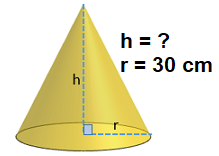We know volume of cone is given by,

Volume = πr2h/3

⇒ 3×Volume = πr2h

⇒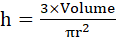⇒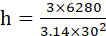⇒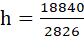⇒ h = 6.67

Thus, perpendicular height of cone is 6.67 cm.

Question 6.

Surface area of a cone is 188.4 sq.cm and its slant height is 10cm. Find its perpendicular height (π = 3.14)

Given: Surface area of cone (SA) = 188.4 cm2

Slant height of cone (l) = 10 cm

So, we need to write the formula of curved surface area of cone with slant height l,

Surface Area = πrl

Where, r = radius of base of cone

Substituting values in the formula, we get

188.4 = 3.14×r×10

⇒ 188.4 = 31.4r

⇒ r = 188.4/31.4

⇒ r = 6

So we have,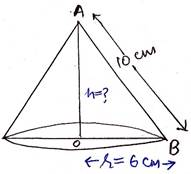We need to find the perpendicular height, h.

Using Pythagoras theorem in ∆AOB, we get

AB2 = AO2 + OB2

⇒ AO2 = AB2 – OB2

⇒ h2 = 102 – 62

⇒ h2 = 100 – 36 = 64

⇒ h = √64 = 8

Thus, perpendicular height of the cone is 8 cm.

Question 7.

Volume of a cone is 1212 cm3 and its height is 24cm. Find the surface area of the cone.

(π = 22/7)

Given: Volume of cone (V) = 1212 cm3

Height of cone (h) = 24 cm

We know the formula of volume of cone,

Volume = πr2h /3

⇒ 3V = πr2h

⇒ r2 =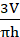⇒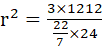⇒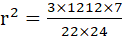⇒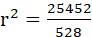⇒ r2 = 48.20 …(i)

⇒ r = √48.20 = 6.94

⇒ r ≈ 7 …(ii)

We know,

l = √(r2 + h2)

⇒ l = √(48.20 + 242) [from (i)]

⇒ l = √(48.20 + 576)

⇒ l = 24.98

⇒ l ≈ 25 …(iii)

⇒ Slant height of cone is 25 cm.

Curved surface area of cone is given by,

CSA = πrl

⇒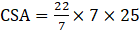[from (ii) and (iii)]

⇒ CSA = 550 …(iv)

Thus, curved surface area of cone is 550 cm2.

Question 8.

The curved surface area of a cone is 2200 sq.cm and its slant height is 50 cm. Find the total surface area of cone.

(π = 22/7)

Given: Curved surface area of cone (CSA) = 2200 cm2

Slant height of cone (l) = 50 cm

For total surface area, we have the formula

TSA = πrl + πr2

⇒ TSA = CSA + πr2 [∵, Curved surface area (CSA) = πrl] …(i)

Where, r = radius of the base of the cone

We have CSA, we just need to find radius of the base of the cone (r).

So, we know

CSA = πrl

Substituting values in above equation,

⇒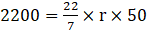⇒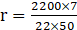⇒ r = 14

⇒ Radius of the base of the cone = 14 cm

Thus, substituting value of CSA and r in equation (i),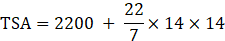⇒ TSA = 2200 + 616

⇒ TSA = 2816

Thus, total surface area of cone is 2816 cm2.

Question 9.

There are 25 persons in a tent which is conical in shape. Every person needs an area of 4 sq.m. of the ground inside the tent. If height of the tent is 18m, find the volume of the tent.

Given that, the tent is conical in shape.

If 1 person occupies area inside the tent = 4 m2

Then 25 people will occupy area inside the tent = 25 × 4 = 100 m2

⇒ Base area of the cone = 100 m2 …(i)

Also, base area of the cone is given by,

Base area of the cone = πr2

⇒ 100 = πr2 [from (i)]

⇒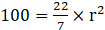⇒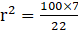⇒ r2 = 31.82 …(ii)

⇒ r = 5.64

We have, radius of the base of the cone (r) = 5.64 m

Height of the conical tent (h) = 18 m

Now we need to find volume of this conical tent. The formula is:

Volume = πr2h/3

Substituting values, we get

Volume = (3.14 × 31.82 × 18)/3 [from (ii)]

⇒ Volume = 599.5

⇒ Volume ≈ 600 m3

Thus, we can say volume of the conical tent is approximately 600 m3.

We have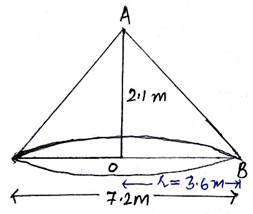Given: height of the cone (h) = 2.1 m

Diameter of the base of cone (d) = 7.2 m

⇒ Radius of the base of cone (r) = 7.2/2 = 3.6 m

Volume (V) of the conical fodder is given by,

Volume = πr2h/3

⇒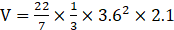⇒ V = 28.512

Thus, volume of cone is 28.512 m3.

If it is covered by polythene in rainy season, then it will be covered on the curved surface area, not on the base.

Curved surface area (CSA) of cone is given by,

CSA = πrl …(i)

We need to find l.

In ∆AOB, using Pythagoras theorem,

AB2 = AO2 + OB2

⇒ l2 = 2.12 + 3.62

⇒ l2 = 4.41 + 12.96

⇒ l2 = 17.37

⇒ l = √17.37 = 4.17 [given that √17.37 = 4.17]

Substituting l = 4.17 in equation (i),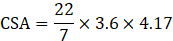⇒ CSA = 47.18

Thus, curved surface area of cone is 47.18 m2.

Hence, volume of cone is 28.51 m3 and curved surface area of cone is 47.18 m2.

###### Practice Set 9.3
Question 1.

Find the surface areas and volumes of spheres of the following radii.

i. 4 cm ii. 9 cm

iii. 3.5 cm. (π = 3.14)

(i). When radius (r) = 4 cm

Surface area of the sphere is given by,

Surface area = 4πr2

⇒ Surface area = 4 × 3.14 × 42

⇒ Surface area = 200.96

Volume of the sphere is given by,

Volume =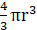⇒ Volume =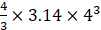⇒ Volume = 267.95

Thus, surface area of sphere is 200.96 cm2 and volume of sphere is 267.95 cm3.

(ii). When radius (r) = 9 cm

Surface area of the sphere is given by,

Surface area = 4πr2

⇒ Surface area = 4 × 3.14 × 92

⇒ Surface area = 1017.36

Volume of the sphere is given by,

Volume =⇒ Volume =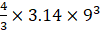⇒ Volume = 3052.08

Thus, surface area of sphere is 1018.29 cm2 and volume of sphere is 3052.08 cm3.

(iii). When radius (r) = 3.5 cm

Surface area of the sphere is given by,

Surface area = 4πr2

⇒ Surface area = 4 × 3.14 × 3.52

⇒ Surface area = 153.86

Volume of the sphere is given by,

Volume =⇒ Volume =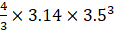⇒ Volume = 179.50

Thus, surface area of sphere is 153.86 cm2 and volume of sphere is 179.50 cm3.

Question 2.

If the radius of a solid hemisphere is 5cm, then find its curved surface area and total surface area. (π = 3.14)

Given: Radius of hemisphere = 5 cm

Curved surface area is given by,

Curved surface area = 2πr2

= 2 × 3.14 × 52

= 157

Total surface area is given by,

Total surface area = 3πr2

= 3 × 3.14 × 52

= 235.5

Thus, curved surface area of hemisphere is 157 cm2 and total surface area of hemisphere is 235.5 cm2.

Question 3.

If the surface area of a sphere is 2826 cm2 then find its volume. (π = 3.14)

Surface area of sphere = 2826 cm2

Surface area of sphere is given by,

Surface area = 4πr2

Substituting the values in above equation,

⇒ 2826 = 4 × 3.14 × r2

⇒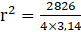⇒ r2 = 225

⇒ r = √225

⇒ r = 15

Volume of sphere is given by,

Volume =⇒ Volume =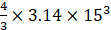⇒ Volume = 14130

Thus, volume of sphere is 14130 cm2.

Question 4.

Find the surface area of a sphere, if its volume is 38808 cubic cm. (π = 22/7)

Given: Volume of sphere = 38808 cm3

Volume of sphere is given by,

Volume =Substituting value of volume of sphere in above equation, we get

⇒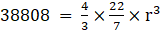⇒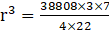⇒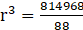⇒ r3 = 9261

⇒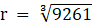⇒ r = 21

Surface area of sphere is given by,

Surface area = 4πr2

⇒ Surface area =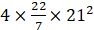= 5544

Thus, surface area of the sphere is 5544 cm2.

Question 5.

Volume of a hemisphere is 18000 p cubic cm. Find its diameter.

Given: Volume of hemisphere = 18000π cm3

And we know volume of hemisphere is given by,

Volume =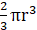Substituting value of volume of hemisphere in above equation, we get

18000π = 2/3 πr3

⇒ 18000 × 3 = 2r3

⇒ r3 = (18000 × 3)/2

⇒ r3 = 9000 × 3

⇒ r3 = 3 × 3 × 3 × 1000

⇒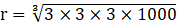⇒ r = 3 × 10

⇒ r = 30

If radius of hemisphere is 30 cm, then diameter of the hemisphere will be twice of the radius.

⇒ diameter = 2(30)

⇒ diameter = 60

Thus, diameter of hemisphere is 60 cm.

###### Problem Set 9
Question 1.

If diameter of a road roller is 0.9 m and its length is 1.4 m, how much area of a field will be pressed in its 500 rotations?

We have the road roller which is cylindrical,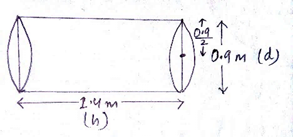Now, given that

Length of the road roller, i.e., height of the cylinder (h) = 1.4 m

Diameter of the road roller = 0.9 m

⇒ Radius of base of the cylindrical road roller (r) = Diameter/2

⇒ Radius (r) = 0.9/2 m

Now, since the road roller is rotating, we can deduce that only the curved surface of the cylindrical road roller will be pressed against the field.

⇒ Area of the field pressed in 1 rotation = Curved surface area of the cylindrical road roller

Then, Area of the field pressed in 500 rotations = 500 (Curved surface area of cylindrical road roller) …(i)

So, curved surface area of cylinder is given by

CSA = 2πrh

Substituting values, we get

CSA =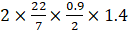⇒ CSA = 3.96

Now, using equation (i) we get

Area of the field pressed in 500 rotations = 500 × 3.96 = 1980 m2

Thus, area of field pressed by the road roller in 500 rotations is 1980 m2.

Question 2.

To make an open fish tank, a glass sheet of 2 mm gauge is used. The outer length, breadth and height of the tank are 60.4 cm, 40.4 cm and 40.2 cm respectively. How much maximum volume of water will be contained in it?

The fish tank is cuboidal.

Although it is open from the top, it won’t affect our calculations for finding volume of the fish tank.

Outer dimensions:

Length (l’) = 60.4 cm

Height (h’) = 40.2 cm

Width of the gauge is width of the tank (w) = 2 mm = 0.2 cm [∵, 1 mm = 10-1 cm]

⇒ 2w = 2 × 0.2 = 0.4 cm

Inner dimensions:

Length (l) = l’ – 2w = 60.4 – 0.4 = 60 cm

Breadth (b) = b’ – 2w = 40.4 – 0.4 = 40 cm

Height (h) = h’ – 2w = 40.2 – 0.4 = 39.8 cm

Volume of the cuboidal fish tank is given by,

Volume = lbh

= 60 × 40 × 39.8

= 95520 cm3

Thus, volume of water that the fish tank will contain is 95520 cm3.

Question 3.

If the ratio of radius of base and height of a cone is 5:12 and its volume is 314 cubic metre. Find its perpendicular height and slant height (π = 3.14)

Given: Volume of cone = 314 m3

Radius of base of cone: height of cone = r : h

= 5 : 12

Let radius of base of cone (r) = 5x

And let height of cone (h) = 12x

Volume of cone is given by,

Volume = πr2h/3

Substituting values, we get

314 = (3.14 × (5x)2 × 12x)/3

⇒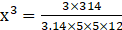⇒ x3 = 1

⇒ x = 1

Thus, radius of the base of cone = 5×1 = 5 m

And height of cone = 12×1 = 12 m

For slant height, we have the relation

l = √(r2 + h2)

⇒ l = √(52 + 122)

⇒ l = √(25 + 144)

⇒ l = √169

⇒ l = 13

Hence, we have got perpendicular height of cone to be 12 m and slant height of the cone to be 13 m.

Question 4.

Find the radius of a sphere if its volume is 904.32 cubic cm. (π = 3.14)

Given: Volume of the sphere = 904.32 cm3

And volume of the sphere is given by,

Volume = 4πr3/3

We have to find radius (r), so by rearranging the above equation, we get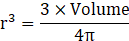Substituting values in the above equation, we get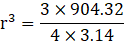⇒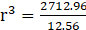⇒ r3 = 216

⇒ r = (216)1/3

⇒ r = 6

Thus, radius of sphere is 6 cm.

Question 5.

Total surface area of a cube is 864 sq.cm. Find its volume.

Given: Total surface area of cube = 864 cm2

And total surface area of cube is given by,

Total surface area = 6(side)2

Substituting values in the above equation,

864 = 6(side)2

⇒ (side)2 = 864/6 = 144

⇒ side = √144

⇒ side = 12

If the length of side of cube is 12 cm, then volume is given by

Volume = (side)3

⇒ Volume = (12)3

⇒ Volume = 1728

Thus, volume of cube is 1728 cm3.

Question 6.

Find the volume of a sphere if its surface area is 154 sq.cm.

Given: surface area of sphere = 154 cm2

And we know surface area of sphere is given by,

Surface area = 4πr2

Substituting values in the above equation, we get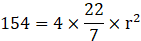⇒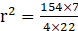⇒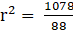⇒ r2 = 12.25

⇒ r = √12.25

⇒ r = 3.5

If radius of sphere is 3.5 cm, then volume can be easily found as the formula of volume of sphere is given by,

Volume = 4πr3/3

⇒ Volume =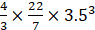⇒ Volume =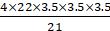⇒ Volume = 179.67

Thus, volume of sphere is 179.67 cm3.

Question 7.

Total surface area of a cone is 616 sq.cm. If the slant height of the cone is three times the radius of its base, find its slant height.

Given: Total surface area of cone = 616 cm2

According to the question,

Slant height of cone = 3 (radius of the base of cone)

Let slant height of cone = l

And let radius of the base of cone = r

Then, l = 3r …(i)

Total surface area of cone is given by,

TSA = πrl + πr2

Substituting TSA = 616 cm2,

616 = πr(3r) + πr2 [Using equation (i)]

⇒ 616 = 3πr2 + πr2

⇒ 616 = 4πr2

⇒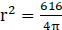⇒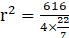⇒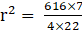⇒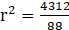⇒ r2 = 49

⇒ r = √49

⇒ r = 7

Putting r = 7 in equation (i), we get

l = 3 × 7 = 21

Thus, slant height of cone is 21 cm.

Question 8.

The inner diameter of a well is 4.20 metre and its depth is 10 metre. Find the inner surface area of the well. Find the cost of plastering it from inside at the rate Rs.52 per sq.m.

Given: A well of cylindrical shape.

Inner diameter = 4.20 m

⇒ Inner radius (r) = 4.20/2 = 2.1 m

Height of well (h) = 10 m

To find inner surface area of the wall, we’ll have to apply curved surface area of cylinder:

CSA = 2πrh

Substituting values in the above equation, we get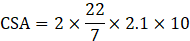⇒ CSA = 132 m2

Plastering of the well will be done on the wall only, so we will be using curved surface area of the well for finding cost of plastering the well.

If cost of plastering 1 m2 = Rs. 52

Then cost of plastering 132 m2 = 132 × 52

= Rs. 6864

Thus, inner surface area of the well is 132 m2 and cost of plastering it is Rs. 6864.

Question 9.

The length of a road roller is 2.1m and its diameter is 1.4m. For levelling ground 500 rotations of the road roller were required. How much area of ground was leveled by the road roller? Find the cost of levelling at the rate of Rs. 7 per sq. m.

The road roller is cylindrical in shape as described.

Given: length of cylindrical road roller (h) = 2.1 m

Diameter of the road roller = 1.4 m

⇒ Radius of the cylindrical road roller (r) = 1.4 /2 = 0.7 m

The figure is given below: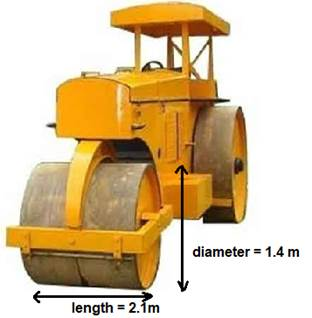When the cylindrical road roller will roll on the ground, the only portion of the cylinder that will touch the ground is the curved surface of cylindrical road roller.

Thus, if we find curved surface area of cylinder, we can know area of ground levelled.

Curved surface area of cylinder is given by,

CSA = 2πrh

Where h = height of cylinder when placed vertical or length of the cylinder when placed horizontal

Substituting values in above equation, we get

⇒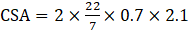⇒ CSA = 9.24

This implies that, area of ground leveled in 1 rotation = 9.24 m2

Then, area of ground leveled in 500 rotations = 9.24 × 500 = 4620 m2

If cost of levelling 7 m2 ground = Rs. 7

Then cost of levelling 4620 m2 ground = 4620 × 7 = Rs. 32340

Thus, area of the ground that was levelled by the road roller is 4620 m2 and cost of levelling it is Rs. 32340.